# Young's modulus, Hooke's law and material properties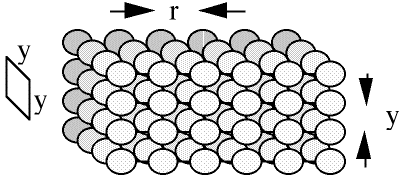Many objects deform according to Hooke's law; many materials behave elastically and have a Young's modulus. In this section, we explain the origin of elastic properties and Hooke's law by consideration of the forces and energies between atoms or molecules in a solid. But first, an experiment.

### An example of Hooke's law and its limitations

This series of photographs shows a spring being stretched and compressed by known forces. The extension or compression can be read on the ruler, and the force is measured with a spring balance (which itself uses a spring).
The data from the experiment shown above are plotted on the graph. Hooke's law is F = -kx where F is the applied force, x is the deformation, and k a constant for a particular spring. Note that Hooke's law is only valid over a limited range: in this case, once the spring is compressed about 10 cm, larger forces produce hardly any further reduction in length, because the spring's coils are in contact.

### Attractive and repulsive forces between atoms

One can deduce quite a bit about intermolecular or interatomic forces just from a simple experiment, and from the observations that solid objects are difficult to compress and, once broken, do not spontaneously repair.

Take a short piece of metal wire (e.g. a straightened paper clip), and try to stretch it along its length. Unless the wire is very thin or you are very strong, the amount of stetching will be small, and the wire will not break. What has happened here is that you have slightly increased the average distance, r, between the atoms. However, the attractive force between pairs of atoms has been able to resist the tensile force you applied.

Now try to shorten the metal by exerting a compressive force along its length. Here, you have slightly decreased the average distance between the atoms but the repulsive force between pairs of atoms has been able to resist the compressive force you applied.

From this you can deduce that, (i) when the interatomic spacing is greater than its unstressed value, the attractive forces between atoms must be greater than the repulsive forces (the attractive forces balance both the repulsive forces and the forces you impose). Conversely, (ii) when the interatomic spacing is less than its unstressed value, the repulsive forces between atoms must be greater than the attractive forces.

Two further observations: First it is extremely difficult to compress a metal, so (iii) the repulsive forces must become very large, even for small reductions in r. Second, once you have broken a piece of metal, it doesn't automatically spring back together: (iv) if the atoms are separated from each other by even a distance of much less than a mm, the attractive forces are effectively zero.

From all these observations, we can deduce that the interatomic repulsive and attractive forces, as a function of interatomic separation must look qualitatively rather as shown in the graph below at left.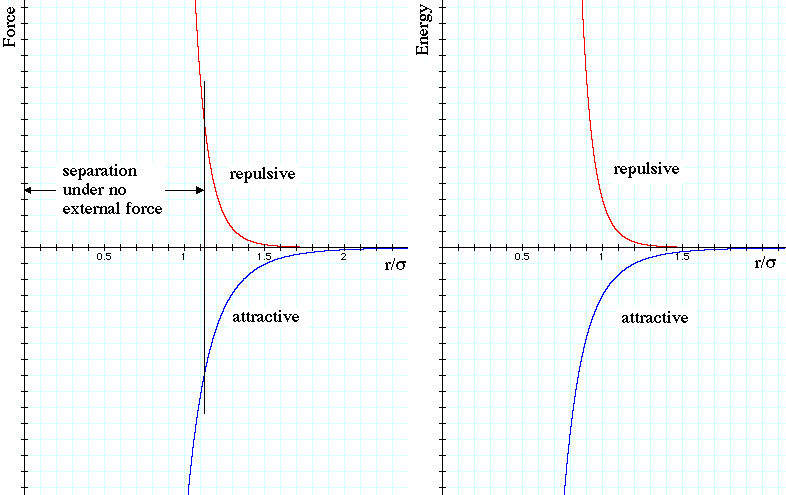By convention, repulsive forces are taken as positive and attractive as negative. (This conveniently makes their potential energies positive and negative respectively, as shown at right, in which the zero of potential energy is taken at large separation.) The vertical grey line marks the separation at which their magnitudes are equal, which identifies the value or r for which there is no externally imposed force. On these graphs, this occurs at r = r0 = 1.12σ, and the significance of the characteristic length σ will be explained later. Attraction is stronger than repulsion under tension (to the right of the vertical line, r > 1.12σ) and weaker than repulsion under compression. Note too that, as our observations above require, the repulsive force rises very rapidly with compression (r < 1.12σ) and that both forces go rapidly to zero as separation increases.

The division of forces into attractive and repulsive components is at least a little arbitrary: What is really important are the total force and energy, which we can actually measure. This is shown by the purple curve in the next version of these graphs.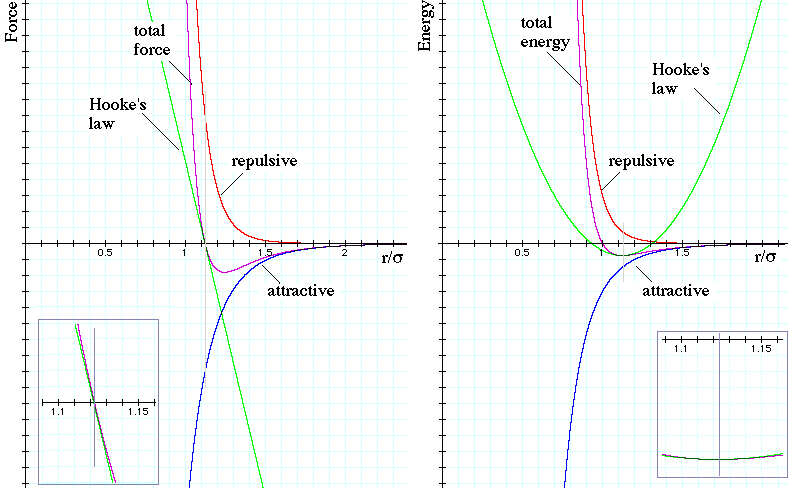Our aim here is to relate these curves to Young's modulus and Hooke's law. But how can curves like these give a linear relation between the force applied and the stretching of the interatomic bonds? The answer is that Hooke's law only applies over a limited range of deformation. It corresponds to a linear approximation to F(r), passing through the point (r0,0), where r0 is the unstressed length. Integrating Hooke's law gives a potential energy U(r) that is parabolic about the minimu at r0. Hooke's law thus corresponds to the green linedrawn on the F(r) plot and the green parabola on the U(r) plot. The insets on each graph show close-ups of these approximation, and show that the approximations are poor for deformations of more than a few percent.

(While discussing this graph, let's observe that, although the Hooke's law approximation for U(r) is symmetrical about its minimum, these curves (and more realistic curves) are not. This asymmetry about the minimum in U(r) is responsible for the thermal expansion of (most) condensed phases: At finite temperatures, the atoms are not found exactly at the minimum of U, because they have finite kinetic energy due to their thermal motion. Oversimplifying in the interests of brevity, we can argue that the average value of U at a value above the minimum lies to the right of the minimum, and increases as more thermal energy is added.)

### Deriving Young's modulus from atomic forces

The curves shown above are simple polynomials. They are analytical approximations to realistic interatomic forces, but will illustrate how to relate atomic properties to macroscopic material properties. Consider the potential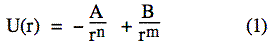where A, B, n and m are constants that we shall discuss below. Remembering that dU  =  − dW  ≡  − F.ds  gives us F - dU/dr, so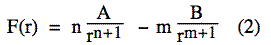As discussed above, the minimum in U occurs when F = 0. Lets call this value of separation r0. Using (2) and rearranging: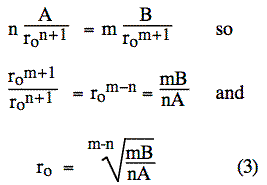We can substitute this value into (1) to get the minimum value U(r0). (In a diatomic molecule, this value would give an estimate of the binding energy but, as it's more complicated for a crystal, we'll leave that calculation aside.)

The sketch shows a simple model of a crystalline solid. In the horizontal (x) axis, the interatomic separation is r. In both perpendicular directions, the separation is y, as shown in this sketch.

Young's modulus Y for a material is defined as the ratio of tensile stress to tensile strain. The tensile stress is the force per unit area F/A, and the strain is the proportional increase in length parallel to the applied force δx/x. If we divide the area elements of A = y2, we have one intermolecular bond and its force F to consider.So the definition of Young's modulus givesTaking the derivative of (2) gives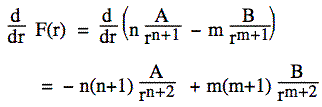Substituting  r = r0from (3) and using the defintion of Y:where A, n and m are the constants in equation (1), r0 and y are the inter-atomic separations in the longitudinal and transverse directions.

The potential U(r) that I have used to plot the curves above is the Lennard-Jones potential

U(r)  =  4ε[(σ/r)12 − (σ/r)6]

which is why the characteristic length σ was used in the previous plots. It may be argued that the r−6 term represents retarded van der Waals forces. However, the repulsive term is simply a convenient, differentiable function that gives a very strong repulsion. Substitution in (3) gives r0 = 21/6σ = 1.12σ, whence the value quoted above.

So, we have related Young's modulus to individual atomic forces and energies: the m, n, A and B in the more general empirical form or, by subtitution, the ε and σ in the Lennard-Jones potential (set A = 4εσ6, B = 4εσ12, n = 6 and m = 12). To relate Hooke's law to Young's modulus in the experiment above, it would be necessary to consider bending of the wire. In bending, one side of an object is stretched and the other compressed. This also requires considering the geometry of the spring. Although the length of the spring may change by many percent, nowhere is the steel compressed or stretched more than one percent.

### Polymers and entropic forces

Many polymeric materials, such as rubber, have much lower values of Young's modulus than other solids. The stretching mechanism here is different, because to a large extent stretching straightens polymer molecules, rather than changing the average distance between them.

To analyse this, one must consider not just the potential energy, but the free energy, U − TS, where T is the (absolute) temperature and S the entropy. A completely straightened polymer molecule has only one possible configuration. A bent (and therefore shorter) molecule has at least one kink in it. The kinks can occur at many different locations, so usually there are many possible configurations corresponding to a particular shortened length. Consequently, the entropy of the contracted state is higher than that of the straightened state. When you stretch rubber, much of the work you do goes into the −TS term of the free energy, so this is an entropic force.

(More on thermal properties: When the temperature increases, the −TS makes a larger contribution to the free energy, and more molecules are found with more kinks, and are therefore shorter. As a consequence, polymers often contract as the temperature rises, rather than expand, as explained for 'normal' solids above. See Fig 4 in Wolfe, J. (2015) "Cellular thermodynamics: the molecular and macroscopic views" Encyclopedia of Life Sciences. )This work is licensed under a Creative Commons License.